Processing ......FreeComputerBooks.com Links to Free Computer, Mathematics, Technical Books all over the World

Operations Research (OR), Optimization, Linear Programming, Approximation, etc.
Related Book Categories:
 Game TheoryGraph Theory Discrete and Finite Mathematics AI and Logic Programming Numerical Analysis and Computation Statistics and SAS Programming Machine Learning Applied Mathematics Algorithms and Data Structures Financial Mathematics and Engineering Computational and Algorithmic Mathematics Probability and Stochastic Processes
•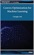Convex Optimization for Machine Learning (Changho Suh)

This book covers an introduction to convex optimization, one of the powerful and tractable optimization problems that can be efficiently solved on a computer. The goal is to help develop a sense of what convex optimization is, and how it can be used.

•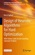Design of Heuristic Algorithms for Hard Optimization

This open access book demonstrates all the steps required to design heuristic algorithms for difficult optimization. The classic problem of the travelling salesman is used as a common thread to illustrate all the techniques discussed.

•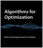Algorithms for Optimization (Mykel J. Kochenderfer, et al.)

A comprehensive introduction to optimization with a focus on practical algorithms for the design of engineering systems. Readers will learn about computational approaches for a range of challenges.

•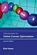Introduction to Online Convex Optimization (Elad Hazan)

This book presents a robust machine learning approach that contains elements of mathematical optimization, game theory, and learning theory: an optimization method that learns from experience as more aspects of the problem are observed.

•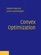Convex Optimization (Stephen Boyd, et al.)

On recognizing convex optimization problems and then finding the most appropriate technique for solving them. It contains many worked examples in fields such as engineering, computer science, mathematics, statistics, finance, and economics.

•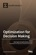Optimization for Decision Making: Linear and Quadratic Models

This book illustrates how to formulate real world problems using linear and quadratic models; It focus on developing modeling skills to support valid decision making for complex real world problems.

•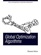Global Optimization Algorithms - Theory and Application

This book is devoted to global optimization algorithms, which are methods to find optimal solutions for given problems. It especially focuses on Evolutionary Computation by discussing evolutionary algorithms, genetic algorithms, Genetic Programming, etc.

•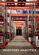Inventory Analytics: A Practicable, Python-Driven Approach

This book provides a comprehensive and accessible introduction to the theory and practice of Inventory Control - a significant research area central to supply chain planning. It adopts a practicable, Python-driven approach to illustrating theories and concepts.

•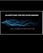Algorithms for Decision Making (Mykel Kochenderfer, et al)

This textbook provides a broad introduction to algorithms for decision making under uncertainty, covering the underlying mathematical problem formulations and the algorithms for solving them.

•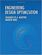Engineering Design Optimization (Joaquim R. Martins, et al)

The philosophy of this book is to provide a detailed enough explanation and analysis of optimization methods so that readers can implement a basic working version. Practical tips are included for common issues encountered in practical engineering design optimization.

•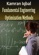Fundamental Engineering Optimization Methods (Kamran Iqbal)

This bookcovers the fundamentals of commonly used optimization methods in engineering design. These include graphical optimization, linear and nonlinear programming, numerical optimization, and discrete optimization.

•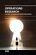Operations Research - the Art of Making Good Decisions

This book is dedicated to operations research of broad applications, it provides a tool for efficient use of natural resources. Both theory and practice of operations research and its related concepts are covered in the book.

•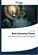Basic Queueing Theory: System Performance Modeling

Queueing Theory is one of the most commonly used mathematical tool for the performance evaluation of systems. The aim of the book is to present the basic methods, approaches in a Markovian level for the analysis of not too complicated systems.

•Knapsack Problems: Algorithms and Computer Implementations

The text fully develops an algorithmic approach to Knapsack Problems without losing mathematical rigor. It provides a comprehensive overview of the methods for solving Knapsack Problems (KP), its variants and generalizations.

•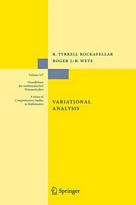Variational Analysis (R. Tyrrell Rockafellar, et al)

This book develops a unified framework and, in finite dimension, provides a detailed exposition of variational geometry and subdifferential calculus in their current forms beyond classical and convex analysis.

•Reinforcement Learning and Optimal Control (Dimitri Bertsekas)

The purpose of the book is to consider large and challenging multistage decision problems, which can be solved in principle by dynamic programming and optimal control, but their exact solution is computationally intractable.

•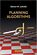Planning Algorithms (Steven M. LaValle)

This is the only book for teaching and referencing of Planning Algorithms in applications including robotics, computational biology, computer graphics, manufacturing, aerospace applications and medicine, etc.

•The Design of Approximation Algorithms (D. P. Williamson)

This book shows how to design approximation algorithms: efficient algorithms that find provably near-optimal solutions. is organized around central algorithmic techniques for designing approximation algorithms, including greedy and local search algorithms, dynamic programming, linear and semidefinite programming, and randomization.

•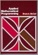Applied Mathematical Programming Using Algebraic Systems

This book is both a reference guide and a text for a course on Applied Mathematical Programming. The material presented will concentrate upon conceptual issues, problem formulation, computerized problem solution, and results interpretation.

•Applied Mathematical Programming (Man-Keun Kim, et al)

It emphasize model-formulation and model-building skills as well as interpretation of computer software output. Focusing on deterministic models, this book is designed for the operations research sequence with a strong computer orientation skills.

•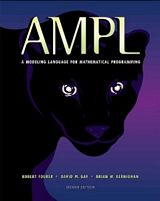AMPL: A Modeling Language for Mathematical Programming

This book is a complete guide to AMPL for modelers at all levels of experience. It begins with a tutorial on widely used linear programming models, and presents all of AMPL's features for linear programming with extensive examples.

•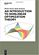An Introduction to Nonlinear Optimization Theory (Marius Durea)

The goal of this book is to present the main ideas and techniques in the field of continuous smooth and nonsmooth optimization. It repeated insights into ideas that are subsequently dealt with and illustrated in detail.

•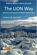The LION Way: Machine Learning Plus Intelligent Optimization

This book captures the state of the art of the interaction between optimization and machine learning in a way that is accessible to people in both fields. Optimization approaches have enjoyed prominence in machine learning.

•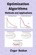Optimization Algorithms- Methods and Applications

This book covers state-of-the-art optimization methods and their applications in wide range especially for researchers and practitioners who wish to improve their knowledge in this field.

•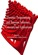Dynamic Programming and Bayesian Inference, Concepts/Apps

This is a book on the far-ranging algorithmic methododogy of Dynamic Programming. It presents a comprehensive and rigorous treatment of dynamic programming, and provides some applications of Bayesian optimization and dynamic programming.

•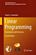Linear Programming: Foundations and Extensions (R. Vanderbei)

This book introduces the theory and applications in optimization. It emphasizes constrained optimization, beginning with a substantial treatment of linear programming and then proceeding to convex analysis, network flows, integer programming, etc..

•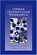Urban Operations Research (Richard C. Larson, et al)

The book covers a wide variety of applied operations research in a way not seen elsewhere. With an emphasis on model building, not theorem proving.

•Methods and Models of Operations Research (Arnold Kaufmann)

This book focuses on the fundamental models and methodologies underlying the practice of Operations Research.

•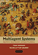Multiagent Systems: Algorithmic, Game-Theoretic, and Logic, etc.

This comprehensive introduction to a burgeoning field is written from a computer science perspective, while bringing together ideas from operations research, game theory, economics, logic, and even philosophy and linguistics.

•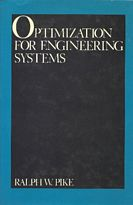Optimization for Engineering Systems (Ralph W. Pike)

This book stands at the interface of mathematics and industrial applications of optimization. The topics were selected for their breadth of application to the optimization of engineering systems, especially continuous ones.

•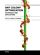Ant Colony Optimization - Techniques and Applications

The book first describes the translation of observed ant behavior into working optimization algorithms. The Ant Colony Optimization is then introduced and viewed in the general context of combinatorial optimization.

•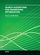Search Algorithms for Engineering Optimization

Heuristic Search is an important sub-discipline of optimization theory. This book explores a variety of applications for search methods and techniques in different fields of electrical engineering. By organizing relevant results and apps.

•Network Calculus: Deterministic Queuing Systems for the Internet

Network Calculus is a set of recent developments that provide deep insights into flow problems encountered in the Internet and in intranets. It shows how it can be applied to the Internet to obtain results that have physical interpretations of practical importance.

•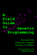A Field Guide to Genetic Programming (Riccardo Poli, et al)

This book provides a complete and coherent review of the theory of Genetic Programming (GP), written by three of the most active scientists in GP. GP solves problems without the user having to know or specify the form or structure of solutions in advance.

•Genetic Algorithms in Applications (Rustem Popa)

This well-organized book takes the reader through the new and rapidly expanding field of genetic algorithms step by step, from a discussion of numerical optimization, to a survey of current extensions to genetic algorithms and applications.

•Discrete-Event Control of Stochastic Networks (Eitan Altman, et al)

Opening new directions in research in both discrete event dynamic systems as well as in stochastic control, this volume focuses on a wide class of control and of optimization problems over sequences of integer numbers.

•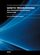Genetic Programming - New Approaches & Successful Applications

The purpose of this book is to show recent advances in the field of Genetic programming, both the development of new theoretical approaches and the emergence of applications that have successfully solved different real world problems.

•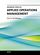Advanced Topics in Applied Operations Management (Y. Holtzman)

This book creatively demonstrates a valuable connection among operations strategy, operations management, operations research, and various departments, systems, and practices throughout an organization.

•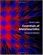Essentials of Metaheuristics (Sean Luke)

This book is an open set of lecture notes on metaheuristics algorithms, intended for undergraduate students, practitioners, programmers, and other non-experts. It covers a wide range of algorithms, representations, selection and modification operators, and related topics, and includes 71 figures and 135 algorithms great and small.

•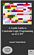A Gentle Guide to Constraint Logic Programming, 3rd Edition

This is an introductory and down-to-earth presentation of Constraint Logic Programming (CLP), for solving combinatorial as well as continuous constraint satisfaction problems and constraint optimization problems.

•Evolutionary Algorithms (Eisuke Kita)

The goal of this book is to provide effective evolutionary algorithms that have been used as an experimental framework within biological evolution and natural selection in the field of artificial life.

•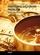Traveling Salesman Problem, Theory and Applications

This book is a collection of current research in the application of evolutionary algorithms and other optimal algorithms to solving the Travelling Salesman Problem (TSP). Most importantly, it presents both theoretical as well as practical applications of TSP,

•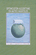Optimization Algorithms on Matrix Manifolds (P.-A. Absil, et al)

This book offers techniques with broad applications in linear algebra, signal processing, data mining, computer vision, and statistical analysis.

•Feedback Systems: An Introduction for Scientists and Engineers

This book provides an introduction to the mathematics needed to model, analyze, and design feedback systems. It uses techniques from physics, computer science, and operations research to introduce control-oriented modeling.

•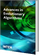Advances in Evolutionary Algorithms (Witold Kosinski)

Provide effective optimization algorithms for solving a broad class of problems quickly, accurately, and reliably by employing evolutionary mechanisms.

•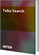Tabu Search (Wassim Jaziri)

The goal of this book is to report original researches on algorithms and applications of Tabu Search to real-world problems as well as recent improvements and extensions on its concepts and algorithms.

•Call Center Optimization: Understanding and Improving

This book gives an overview of the role and potential of mathematical optimization in call centers. It deals extensively with all aspects of workforce management, but also with topics such as call routing and the scheduling of multiple channels.

•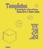Templates for the Solution of Linear Systems: Building Blocks

In this book, which focuses on the use of iterative methods for solving large sparse systems of linear equations, templates are introduced to meet the needs of both the traditional user and the high-performance specialist.

Book Categories
 :All CategoriesTop Free BooksRecent BooksMiscellaneous BooksComputer EngineeringComputer LanguagesComputer ScienceData Science/DatabasesJava and Java EE (J2EE)Linux and UnixMathematicsMicrosoft and .NETMobile ComputingNetworking and CommunicationsSoftware EngineeringSpecial TopicsWeb Programming
Other Categories